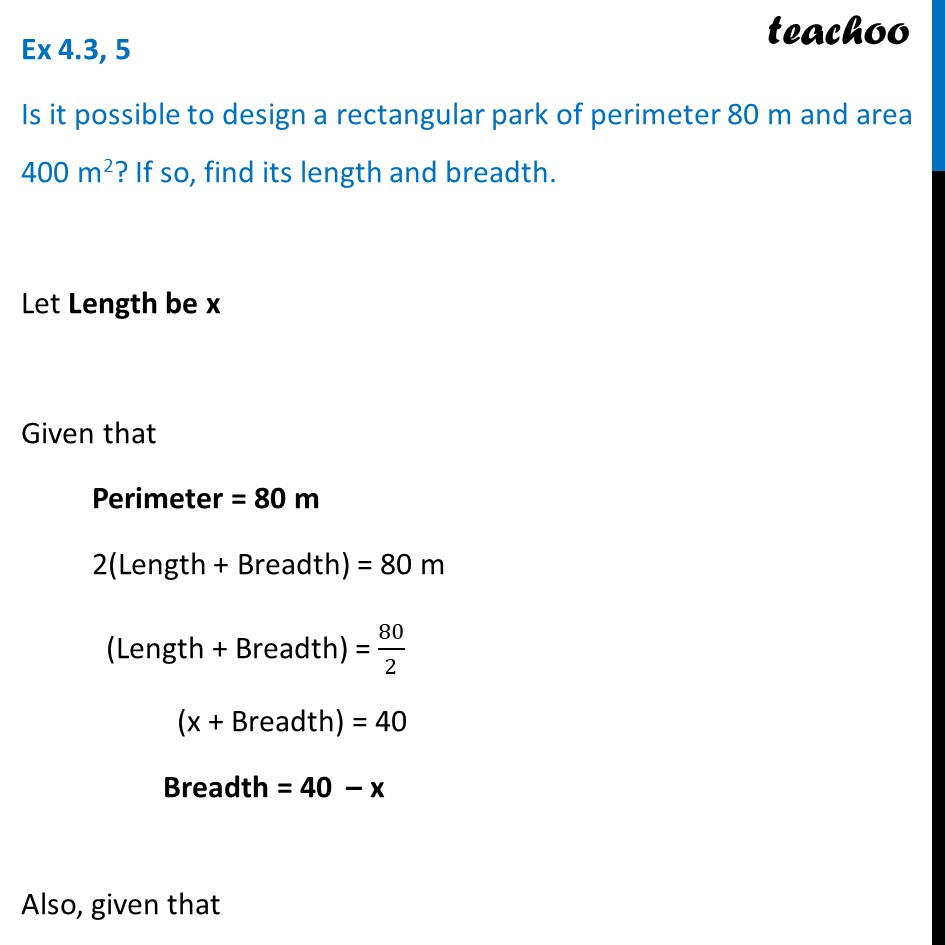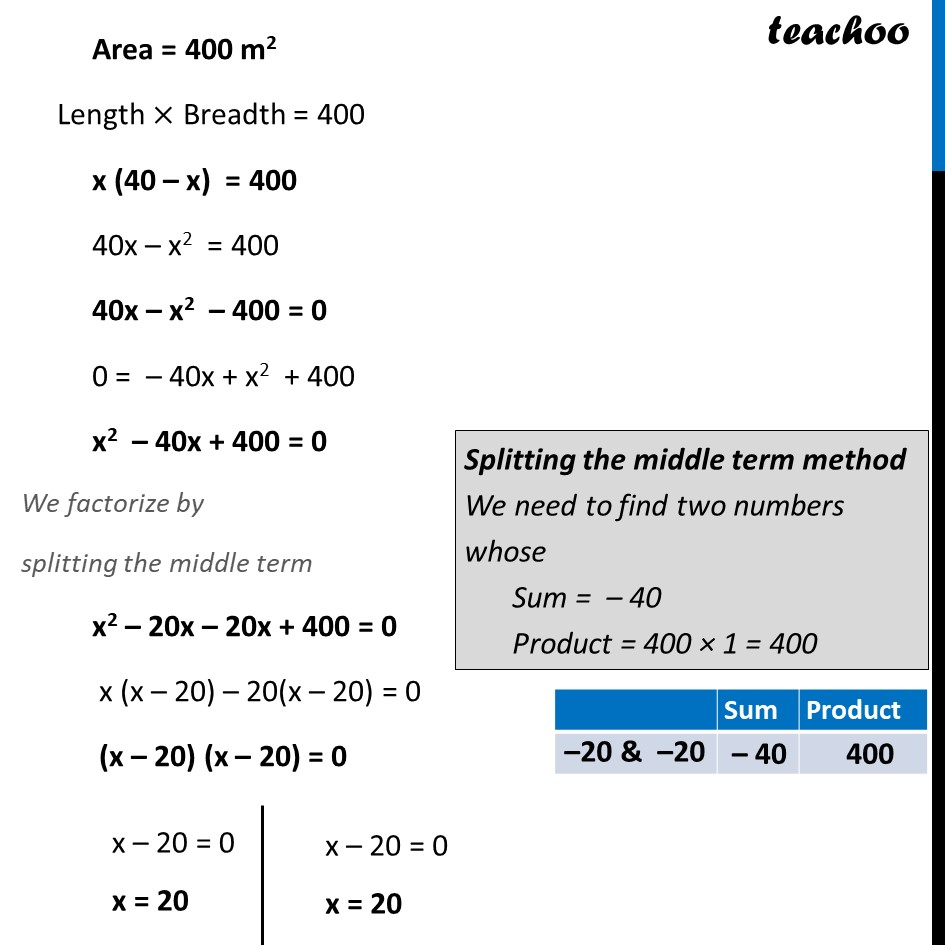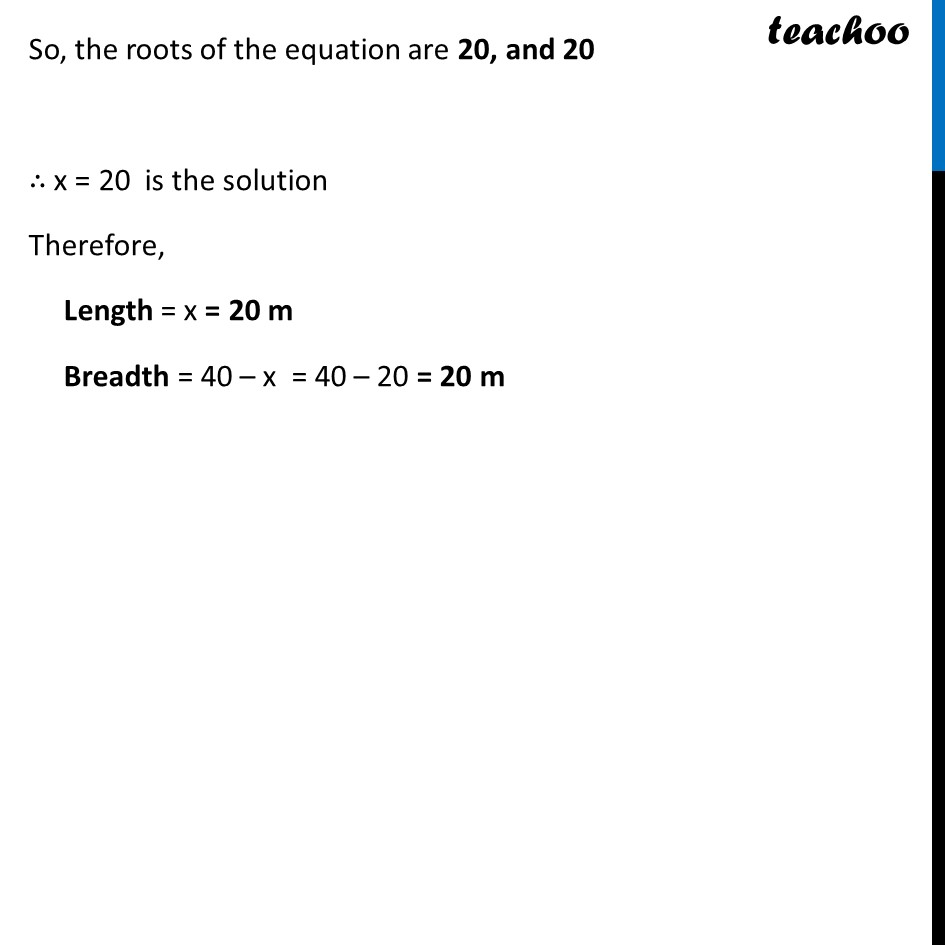Ex 4.3

Chapter 4 Class 10 Quadratic Equations
Serial order wiseLearn in your speed, with individual attention - Teachoo Maths 1-on-1 Class

### Transcript

Ex 4.3, 5 Is it possible to design a rectangular park of perimeter 80 m and area 400 m2? If so, find its length and breadth. Let Length be x Given that Perimeter = 80 m 2(Length + Breadth) = 80 m (Length + Breadth) = 80/2 (x + Breadth) = 40 Breadth = 40 – x Also, given that Area = 400 m2 Length × Breadth = 400 x (40 – x) = 400 40x – x2 = 400 40x – x2 – 400 = 0 0 = – 40x + x2 + 400 x2 – 40x + 400 = 0 We factorize by splitting the middle term x2 – 20x – 20x + 400 = 0 x (x – 20) – 20(x – 20) = 0 (x – 20) (x – 20) = 0 x – 20 = 0 x = 20 x – 20 = 0 x = 20 Splitting the middle term method We need to find two numbers whose Sum = – 40 Product = 400 × 1 = 400 So, the roots of the equation are 20, and 20 ∴ x = 20 is the solution Therefore, Length = x = 20 m Breadth = 40 – x = 40 – 20 = 20 m